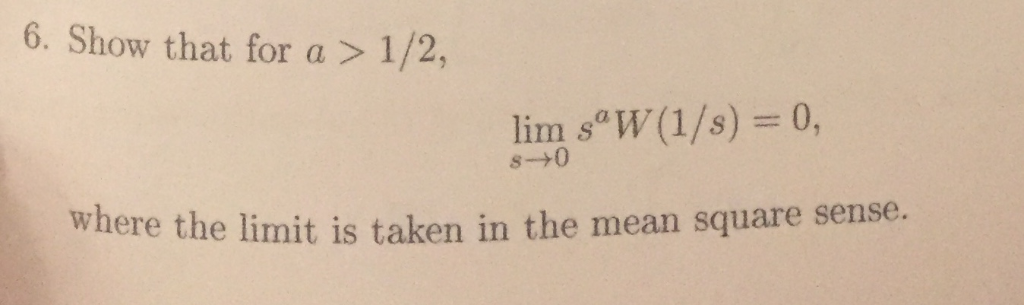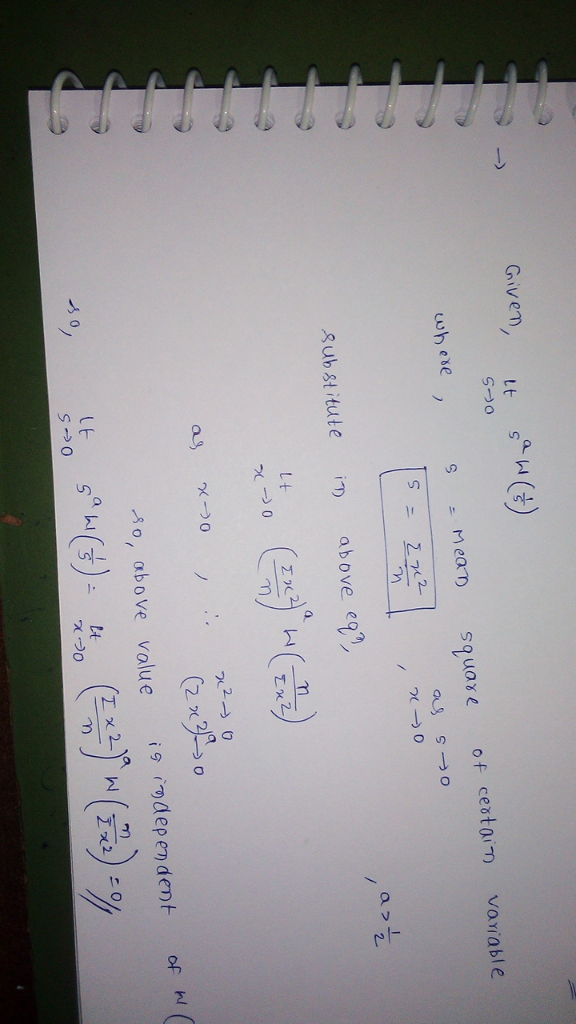# 6. Show that for a > 1/2, lim s W(1/s) s-+0 where the Iimit is taken in the mean square senuse.6. Show that for a > 1/2, lim s W(1/s) s-+0 where the Iimit is taken in the mean square senuse.##### Add Answer of: 6. Show that for a > 1/2, lim s W(1/s) s-+0 where the Iimit is taken in the mean square senuse.
More Homework Help Questions Additional questions in this topic.

• #### If I have ((square root of 13)+(6))^2 would it be factored as -((square root of 13)+(6))((square root of 13)-(6)) or -((square root of 13)+(6))((square root of 13)+(6))

Need Online Homework Help?